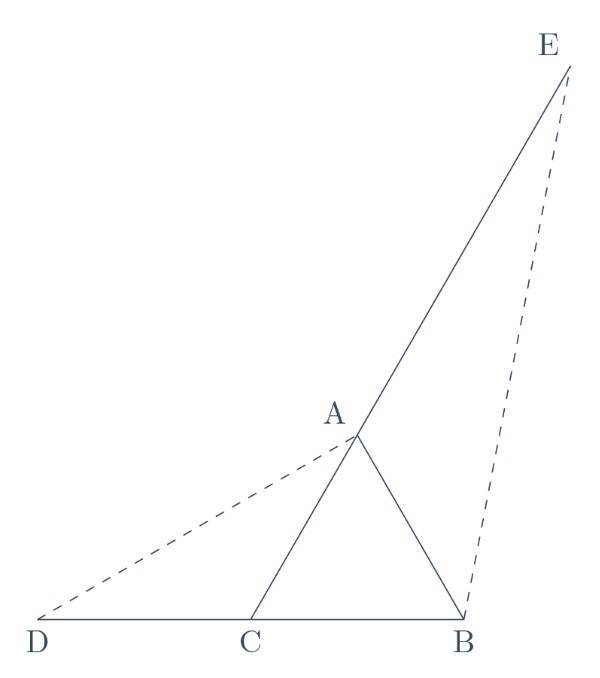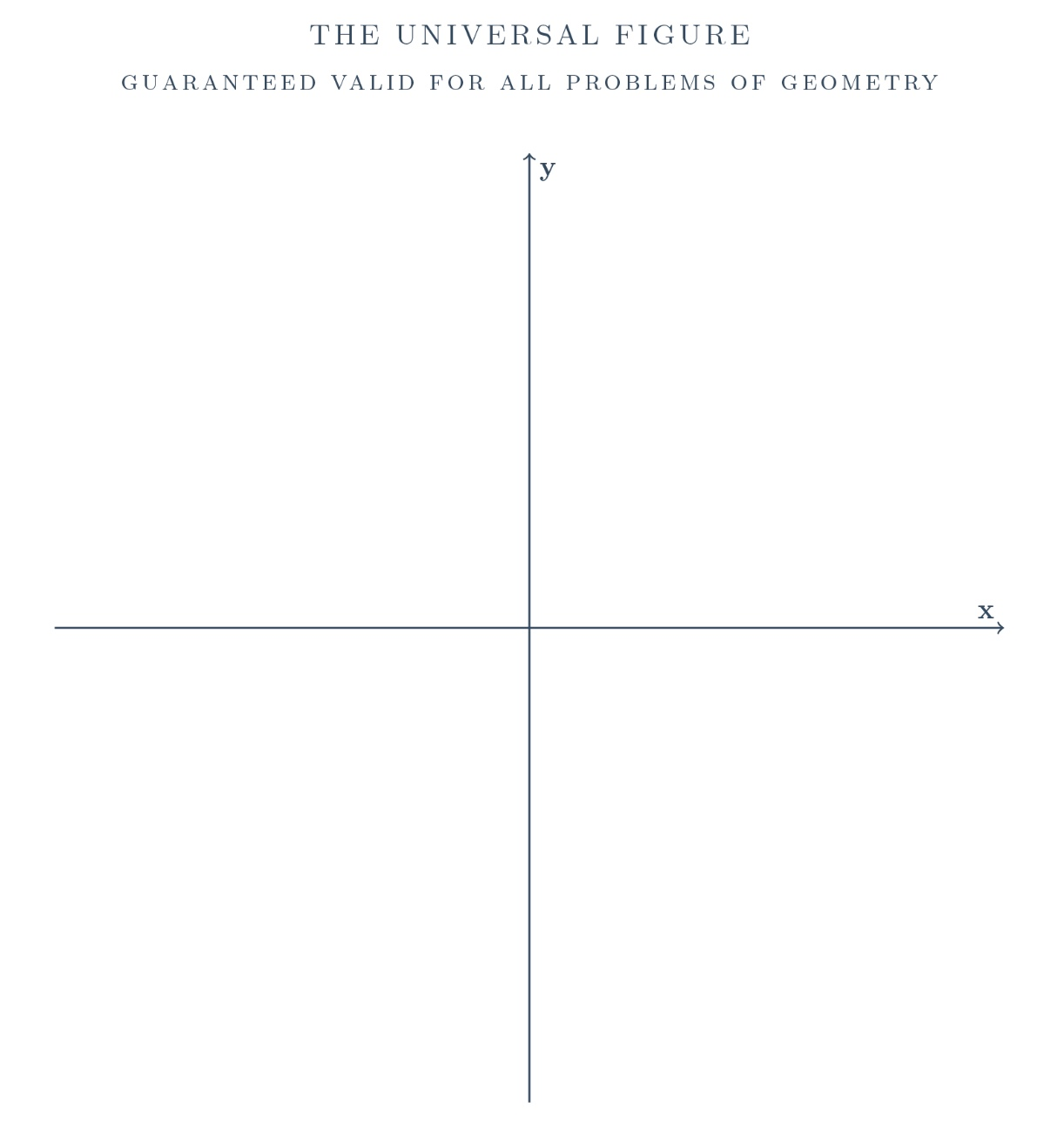# Blog

### Thinking Out Loud – EGMO 2013 Problem 1

by Marcello Mamino

< Go to the previous post in the series

Finally problem 1. Geometry. I'm not going to like it.

EGMO 2013, problem 1. The side $\textrm{BC}$ of the triangle $\textrm{ABC}$ is extended beyond $\textrm{C}$ to $\textrm{D}$ so that $\textrm{CD} = \textrm{BC}$. The side $\textrm{CA}$ is extended beyond $\textrm{A}$ to $\textrm{E}$ so that $\textrm{AE} = 2\textrm{CA}$. Prove that if $\textrm{AD} = \textrm{BE}$, then the triangle $\textrm{ABC}$ is right-angled.Yeah, sure, right-angled. See why I hate the stuff? I don't know where the right angle is. I study my figure, accurately reproduced to the right, with growing discomfort. Somehow, I decide that $\textrm{B}$ and $\textrm{C}$ look wrong, which makes $\textrm{A}$ right, right? I check by Pythagoras' theorem that if $\textrm{A}$ is right, then $\textrm{AD}=\textrm{BE}$.

This problem just screams for Cartesian coordinates. I need to prove that $(x,y):=\textrm{A}$ is on the circle with diameter $\textrm{BC}$. I call $\textrm{B}$ and $\textrm{C}$ respectively $(1,0)$ and $(-1,0)$, so the circle is $x^2+y^2=1$. Point $\textrm{D}$ is $(-3,0)$. Point $\textrm{E}$ is $(3x+2,3y)$. $\displaystyle (x+3)^2 + y^2 =(3x+1)^2 + (3y)^2 \;\Rightarrow\; 8 x^2 + 8 y^2 = 8$.

Average score was 6.14, most contestants obtained the maximum score of 7 points, curiously 9 out of 87 got 5: those that did it with analytic geometry? Number of official solutions: eight. Three by synthetic geometry, plus a variant, two by trigonometry, one by vectors, one by complex numbers, and one by areal coordinates. I'm not kidding, areal coordinates. Seriously, it wasn't so bad, but I don't understand geometry.Alfred Tarski's quantifier elimination method guarantees that all problems of Euclidean geometry can, indeed, be solved algebraically by Cartesian coordinates. Unless you are an extremely fast computer, however, you should not be too excited about the idea of applying Tarski's method in practice: the number of operations required to solve a problem is a tower of exponentials as high as the text of the problem is long (when expressed in a suitable formal language which is, in general, more verbose than English). Tarski's method has been substantially improved by several people, and now computer programs can, indeed, solve geometric problems using these techniques. Yet, unfortunately, such methods are not nearly usable by mere mortals. So, is Tarski's theorem the silver bullet that kills geometry? Of course not. Can I use Tarski's theorem to justify my contempt for the stuff? Definitely.